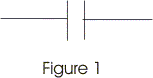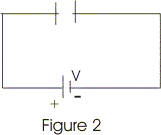# Capacitance And capacitor

## 1. Introduction

• A capacitor (formerly known as condenser) is a device that can store electronic charge and energy.
• All capacitors consists of a combination of two conductors separated by an insulator.
• The insulator is called dielectric which could be oil, air or paper and many more such materials are there wich can act as a dielectric medium between conducting plates of a capacitor.
• Figure 1 below shows the symbol used to represent a capacitor.• Now plates of the capacitor are connected to the terminals of a battery, shown below in figure 2, in order to charge it's conducting plates.• As soon as capacitor is connected to the battery , charge is transferred from one conductor to another.
• Plate connected to positive terminal of the battery becomes positively charged with charge +Q in it and plate connected to negative terminal of the battery becomes negatively charged with charge -Q on it i.e. both plates have equal amount of opposite charge .
• Once the capacitor is fully charged potential difference between the conductors due to their equal and opposite charges becomes equal to the potential difference between the battery terminals.
• For a given capacitor Q∝V and the ratio Q/V is constant for a capacitor.
Thus,
Q=CV                              (1)
where the proportionality constant C is called the capacitance of the capacitor.
• Capacitance of any capacitor depends on shape , size and geometrical arrangement of the conductors.
• When Q is in coulombs (C) and V is in volts(V) then the S.I. unit of capacitance is in farads(F) where
1F=1 coulomb/volt
• One farad is the capacitance of very large capacitor and it's sub-multiples such as microfarad(1μF=10-6) or picofarad(1pF=10-12) are generally used for practical applications.

## 2. Calculation of capacitance

• For calculating capacitance of a capacitor first we need to find the potential difference between it's two conducting plates having charge +Q and -Q.
• For simple arrangements of conductors like two equivalent parallel plates kept at distance d apart or two concentric conducting spheres etc., potential difference can be found first by calculating electric field from gauss's law or by Coulomb's law.
• After calculating electric field , potential difference can be found by integrating electric field using the relation
Va-Vb=∫E.dr
where the limits of integration goes from a to b.
• Once we know the potential difference between two conductors of the capacitor , it's capacitance can be calculated from the relation
C=Q/V                              (2)
• Calculation of capacitance of some simple arrangements would be illustrated in following few articles.